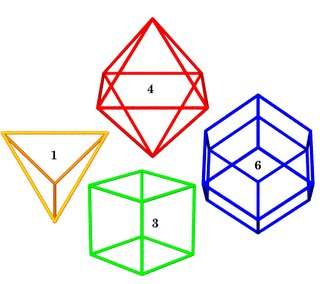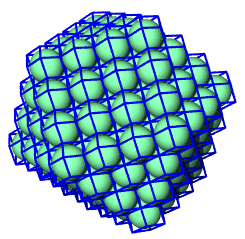## Monday, December 12, 2005

### IQ Test"relative volumes"
(Python + POV-Ray)

• Tetrahedron: fills space with octahedron; inscribes in the cube as face diagonals; self-dual; 24 A mods; same volume as coupler = 8 MITEs (MITE = 2 A mods + 1 B mod).

• Cube: space-filler; inscribes in the rhombic dodecahedron as short face diagonals; dual of octahedron; same volume as six half-couplers (24 MITEs).

• Octahedron: fills space with tetrahedron; inscribes in the rhombic dodecahedron as long face diagonals; dual of cube; 48 A mods + 48 B mods.

• Rhombic Dodecahedron: space-filler; the domain of each ball in the closest-packing arrangement below (CCP); dual of the cuboctahedron; volume of twelve half-couplers (six couplers).3-frequency cuboctahedral packing
(92 + 42 + 12 + 1 = 147 balls)

Followup:
Kirby's address to alumni (Math Forum, Dec 16, 2005)